Groundwater Contaminant Transport. 1-D Step Injection

Groundwater. Chemical injected during a finite time period. Compute concentration as a function of time and distance or back-calculate injected concentration in groundwater. Groundwater advection, dispersion, retardation

Register to enable groundwater "Calculate" button.

 Concentration, C (mg/l): Time, t (day), to compute C: Distance, x (m), to compute C: Check box to include retardation: Injected Concentration, Co (mg/l): Solve for C Solve for Co Duration of Injection, T (day): Clayey Soil (sets K, n, ne, d) Silty Soil (sets K, n, ne, d) Sandy Soil (sets K, n, ne, d) Gravelly Soil (sets K, n, ne, d) User enters K, n, ne, d Dispersivity, a (m): Benzene (sets Koc) Ethyl Benzene (sets Koc) Dichloroethane (sets Koc) Naphthalene (sets Koc) Pyrene (sets Koc) Tetrachloroethylene (sets Koc) Trichloroethylene (TCE) (sets Koc) User enters Koc Diffusion Coeff, D* (m2/s): Hydraulic Cond, K (cm/s): Select Groundwater Units: Hydraulic Grad, -dh/dx (m/m): Concentrations in mg/l or ppm Concentrations in ug/l or ppb Concentrations in kg/m3 Total Porosity, n (%): Times in seconds Times in minutes Times in hours Times in days Times in years Effective Porosity, ne (%): Distance in cm Distance in m Distance in km Distance in inch Distance in feet Distance in miles Soil Bulk Density, d (g/cm3): Dispersivity in mm Dispersivity in cm Dispersivity in m Dispersivity in inch Dispersivity in feet Soil Organic Carbon, foc (%): Diff. and Disp. Coeff. in cm2/s Diff. and Disp. Coeff. in m2/s Diff. and Disp. Coeff. in ft2/s Org. Carbon Coeff, Koc (cm3/g): Hydraulic Conductivity in cm/s Hydraulic Conductivity in m/s Hydraulic Conductivity in m/day Hydraulic Conductivity in ft/s Hydraulic Conductivity in gal/day/ft2 Distribution Coeff, Kd (cm3/g): Hydraulic Gradient in m/m (ft/ft) Hydraulic Gradient in m/km Hydraulic Gradient in inch/mile Hydraulic Gradient in ft/mile Retardation Coeff, Rf: Soil Bulk Density in g/cm3 Soil Bulk Density in kg/m3 Soil Bulk Density in lb/ft3 Pore Water Velocity, Vw (m/s): Koc and Kd in cm3/g Koc and Kd in m3/kg Koc and Kd in ft3/lb Chemical Velocity, Vc (m/s): Velocities in cm/s Velocities in m/s Velocities in m/day Velocities in m/yr Velocities in ft/s Velocities in ft/day Velocities in ft/yr Velocities in mile/hr Dispersion Coeff, D (m2/s): www.LMNOeng.com Peclet Number, Pe=Vc(x/D): © 2015 LMNO Engineering, Plume leading edge, Vct (m): Research, and Software, Ltd. Plume trailing edge, Vc(t-T) (m):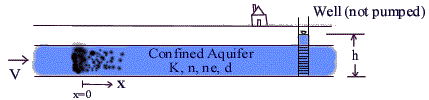Groundwater Units: cm=centimeter, ft=foot, g=gram, gal=U.S. gallon, hr=hour, kg=kilogram, km=kilometer, l=liter, lb=pound, m=meter, mg=milligram, min=minute, mm=millimeter, ppm=part per million (by mass), ppb=part per billion (by mass), s=second, yr=year, ug=microgram

Groundwater topics on this page:   Introduction    Equations    Applications    Variable Definitions   Property Data    Error Messages    References

Groundwater Introduction                       Top of Page
This groundwater calculation simulates one-dimensional (x-direction) transport of a chemical in a confined groundwater aquifer. It is also valid for transport in an unconfined aquifer if the groundwater head gradient (dh/dx) is nearly constant. There are two common boundary conditions for chemical transport: One is a step (i.e. continuous) injection of chemical - the chemical is added at x=0 from time t=0 to t=T. The other common boundary condition is a pulse input where a mass of chemical is added instantaneously at x=0. This groundwater calculator uses the first boundary condition though a pulse input can be simulated by using a short injection time T. The calculator solves for concentration at whatever time and distance is desired by the user.

The groundwater calculation includes advection, dispersion, and retardation. Advection is chemical movement via groundwater flow due to the groundwater hydraulic (i.e. head) gradient. Dispersion is the longitudinal (forward and backward) spreading of the contaminant in groundwater. If there were no dispersion, all of the contaminant would travel at the mean chemical velocity. With dispersion, some chemical travels faster and some slower than the mean velocity; the chemical "spreads out." Retardation causes the mean chemical velocity to be slower than the groundwater velocity. If your chemical exhibits no dispersion, set both the dispersivity (a) and diffusion coefficient (D*) to zero. If the chemical is not retarded, then uncheck the retardation check box or use the chemical drop-down menu to select "User enters Koc" and set Koc =0.0.

Groundwater Equations                           Top of Page

Groundwater Governing Equation and Boundary Conditions
The governing equation for one-dimensional chemical transport in groundwater with advection, dispersion, and retardation is (Van Genuchten and Alves, 1982):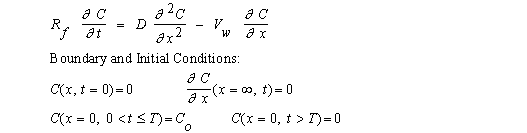Groundwater Solution
The solution to the governing equation and boundary conditions shown above is (Van Genuchten and Alves, 1982):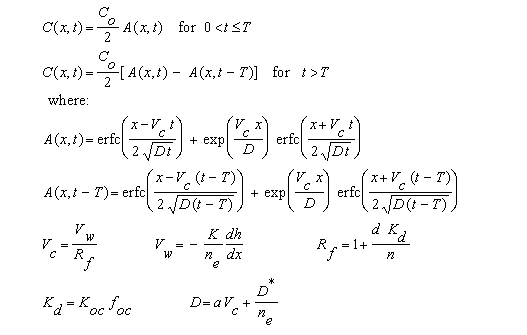erfc( ) is called the "complementary error function."  Our groundwater calculation uses the most accurate numerical representation of erfc( ) given in Abramowitz and Stegun (1972, eqn 7.1.26).

Groundwater Applications                          Top of Page
The following graphs were developed to demonstrate effects of dispersion for trichloroethylene (TCE) in a sandy groundwater aquifer as predicted by the groundwater calculation. The following data were used:
Co = 10,000 mg/l, d = 1.6 g/cm3, dh/dx = -0.007 m/m, D* = 0,   foc = 0.1%,
K = 0.001 cm/s,  Koc = 100 cm3/g, n = 35%, ne = 25%.
Click for groundwater variable definitions
Therefore, Kd = 0.1 cm3/g,  Rf  = 1.46,   Vw = 2.8x10-7 m/s,  and Vc = 1.92x10-7 m/s.

Two injection durations were used:  T=1,000,000 days in Figure 1 and T=100 days in Figure 2.  For Figure 1, T was selected large enough to simulate an infinite duration injection.  In both figures, the concentration front occurs at  x = Vc t = 16.6 m  when  a=0.   In Figure 2, the trailing edge of the concentration front occurs at  x = Vc( t-T) =14.9 m  when a=0.   a is dispersivity.

Figure 1.  TCE concentration profile at 1000 days for an injection of duration 1,000,000 days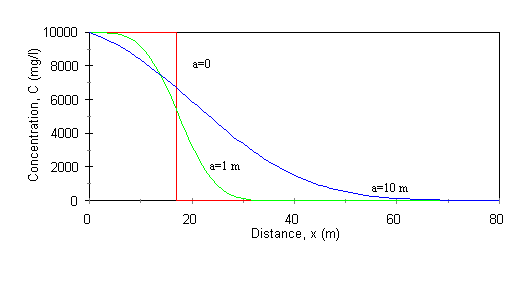Figure 2.  TCE concentration profile at 1000 days for an injection of duration 100 days.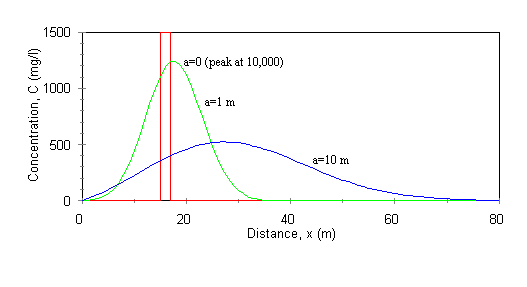Groundwater Variable Definitions  Units: [L]=Length, [M]=Mass, [T]=Time                                 Top of Page
The variables used in the groundwater calculator are:
a = dispersivity [L]. Varies from 0.1 to 100 m. Field and laboratory tests have indicated that a varies with the scale of the test. Large scale groundwater tests have higher a than small lab column tests. An approximate value for a is 0.1 times the scale of your groundwater system (Fetter, 1993). If you are simulating groundwater contaminant transport in a 1 m long laboratory column, then a~0.1 m. However, if you are simulating groundwater transport in a large aquifer greater than 1 km in extent, then use a~100 m.
C = Chemical concentration [M/L3].
Co = Injected concentration at x=0 [M/L3].
d = Dry bulk density of the groundwater aquifer [M/L3].
dh/dx = Groundwater hydraulic (or head) gradient [L/L]. Since dh/dx is negative, we ask you to enter -dh/dx so that you can enter a positive number for convenience. You determine dh/dx from two head measurements using the equation, dh/dx = (h2-h1)/(x2-x1).
D = Dispersion coefficient [L2/T]. The equation D=a Vc + D*/ne is adapted from Ingebritsen and Sanford (1998).
D* = Molecular diffusion coefficient [L2/T]. Varies somewhat for different chemicals but a typical value to use is 1.0x10-9 m2/s (Fetter, 1993).
foc = Organic carbon fraction in soil [%]. (Mass organic carbon per mass soil) x 100%.
K = Hydraulic conductivity of aquifer [L/T].
Kd = Distribution coefficient [L3/M]. Represents chemical partitioning between groundwater and soil.
Koc = Organic carbon partition coefficient [L3/M]. Represents chemical partitioning between organic carbon and water in soil. Good discussion in Lyman et al. (1982).
n = Total porosity of soil [%]. (Void volume/total volume) x 100%.
ne = Effective porosity [%]. Porosity through which flow can occur. A thin film of water bound to soil particles by capillary forces does not move through the groundwater aquifer. ne is always ≤ n.
Pe = Peclet number. Pe=(Vc x ) / D. It is a commonly used dimensionless parameter indicating the relative impact of inertial effects to dispersive effects.
Rf = Retardation factor. Rf =1 if there is no retardation which implies that Vc=Vw. Rf =1 would occur for a conservative tracer; that is, a tracer that does not sorb to the aquifer soil.
t = Time [T]. Time at which C is to be computed.
T = Duration of injection [T]. Co is injected from t=0 to t=T.
Vc = Mean chemical velocity [L/T].
Vw = Pore water velocity [L/T]. Also known as groundwater velocity.
x = Distance [L]. Distance at which to compute C.

Groundwater Property Data                       Top of Page
The following are tables of groundwater hydraulic conductivity, total porosity, effective porosity, bulk density, and organic carbon partition coefficient. Groundwater parameter values have been compiled from a variety of sources such as Coduto (1994), Fetter (1993), Freeze and Cherry (1979), Hillel (1982), and Sanders (1998). The values used in the groundwater calculation above are typical numbers within the ranges given below.

Table of Soil Properties

 Soil Type Hydraulic Conductivity K (cm/s) Total Porosity n (%) Effective Porosity ne (%) Bulk Density d (g/cm3) Clayey 10-9 - 10-6 40-60 0-5 1.2-1.8 Silty 10-7 - 10-3 35-50 3-20 1.1-1.8 Sandy 10-5 - 10-1 20-50 10-35 1.3-1.9 Gravelly 10-1 - 102 25-40 12-30 1.6-2.1

Table of Organic Carbon Partition Coefficient, Koc

 Chemical Koc (cm3/g) Chemical Koc (cm3/g) Benzene 20-400 Pyrene 2000-200,000 Ethyl Benzene 90-1500 Tetrachloroethylene 100-3000 Dichloroethane 10-250 Trichloroethylene 20-500 Naphthalene 100-2500

Error Messages given by groundwater calculation                            Top of Page
"Certain inputs must be > 0."  No groundwater computations. Co , C, d, -dh/dx, K, n, ne, and T must all be > 0 if entered.

"Certain inputs must be ≥ 0."  No groundwater computations. a, D*, foc , Koc, t, and x must all be ≥ 0 if entered.

"n, ne , and foc must be ≤ 100%."  No groundwater computations. Total porosity, effective porosity, and soil organic carbon cannot exceed 100%.

"ne must be ≤ n." No groundwater computations. Effective porosity cannot exceed total porosity.

"Co cannot be determined" or "Co=∞." Co not computed. Certain input combinations result in computing erfc(∞), and erfc(∞)=0.0. Therefore, Co cannot be evaluated or is evaluated as infinity.

"erfc(x,t)=0. Cannot compute Co", "efrc(x,t-T)=0. Cannot compute Co", "A(x,t)=0. Cannot compute Co", or "A(x,t)-A(x,t-T)=0. Cannot compute Co."   Co not computed since division by zero occurs.

Groundwater References                            Top of Page
Abramowitz, M. and I. A. Stegun. 1972. Handbook of Mathematical Functions. Dover Publications, Inc.

Coduto, D. P. 1994. Foundation Design Principles and Practices. Prentice Hall, Inc.

Fetter, C. W. 1993. Contaminant Hydrogeology. Macmillan Pub. Co.

Freeze, R. A. and J. A. Cherry. 1979. Groundwater. Prentice Hall, Inc.

Hillel, D. H. 1982. Introduction to Soil Physics. Academic Press, Inc.

Ingebritsen, S. E. and W. E. Sanford. 1998. Groundwater in Geologic Processes. Cambridge University Press.

Lyman, W. J. Adsorption coefficient for soils and sediments. In Handbook of Chemical Property Estimation Methods. Lyman, W. J., W. F. Reehl, and D. H. Rosenblatt, eds. McGraw-Hill Book Co. 1982. pp. 4-1 thru 4-33.

Sanders, L. L. 1998. A Manual of Field Hydrogeology. Prentice Hall, Inc..

Van Genuchten, M. Th. and W. J. Alves. 1982. Analytical Solutions of the One-Dimensional Convective-Dispersive Solute Transport Equation. United States Department of Agriculture, Agricultural Research Service, Technical Bulletin 1661.

LMNO Engineering, Research, and Software, Ltd.
7860 Angel Ridge Rd.   Athens, Ohio 45701  USA   Phone: (740) 707-2614
LMNO@LMNOeng.com    http://www.LMNOeng.com

To:

Related Calculations:

3-D Slug injection with advection and dispersion